# Least Squares ANOVA ANCOV Least Squares ANOVA Do

• Slides: 17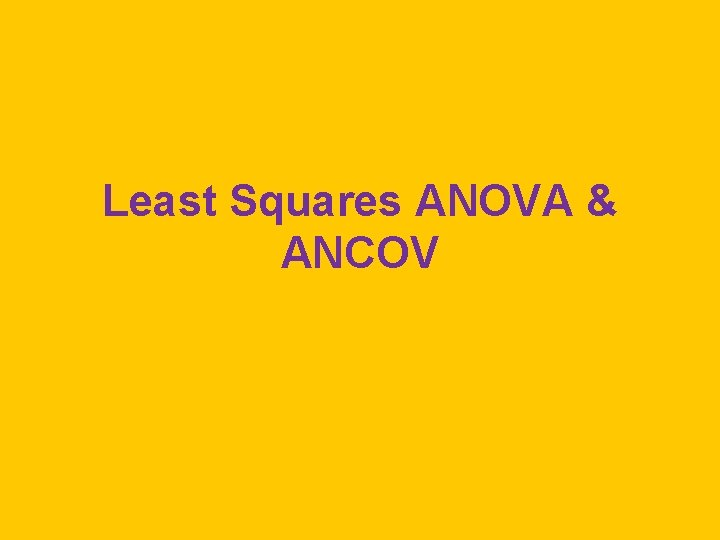Least Squares ANOVA & ANCOV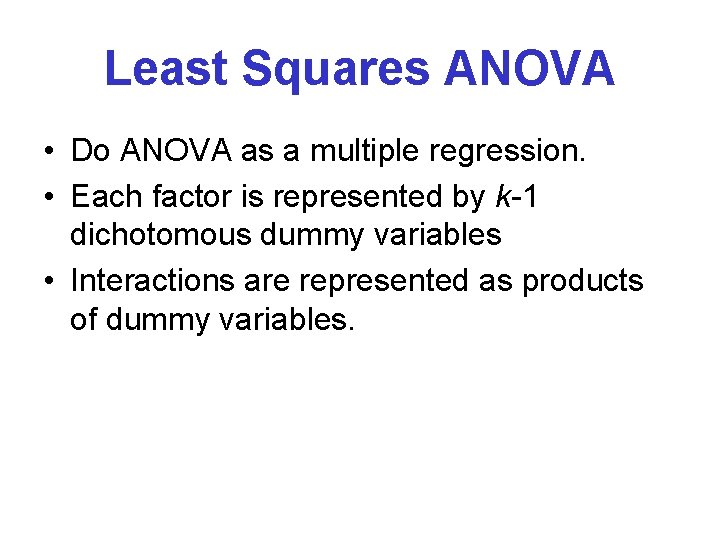Least Squares ANOVA • Do ANOVA as a multiple regression. • Each factor is represented by k-1 dichotomous dummy variables • Interactions are represented as products of dummy variables.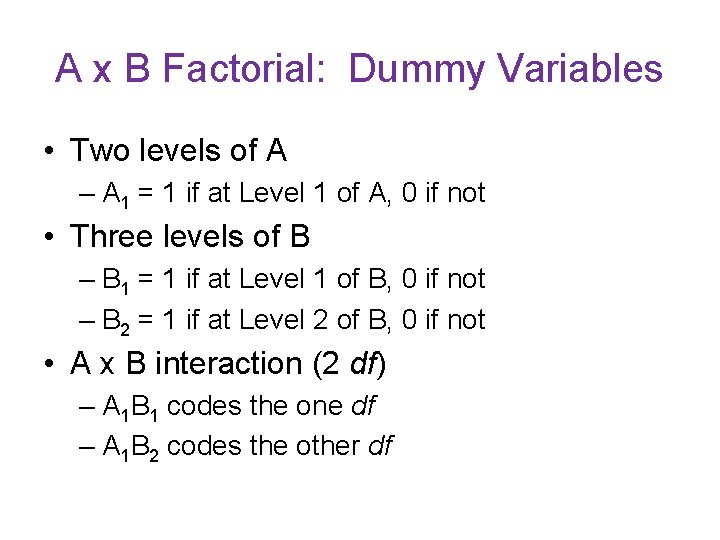A x B Factorial: Dummy Variables • Two levels of A – A 1 = 1 if at Level 1 of A, 0 if not • Three levels of B – B 1 = 1 if at Level 1 of B, 0 if not – B 2 = 1 if at Level 2 of B, 0 if not • A x B interaction (2 df) – A 1 B 1 codes the one df – A 1 B 2 codes the other df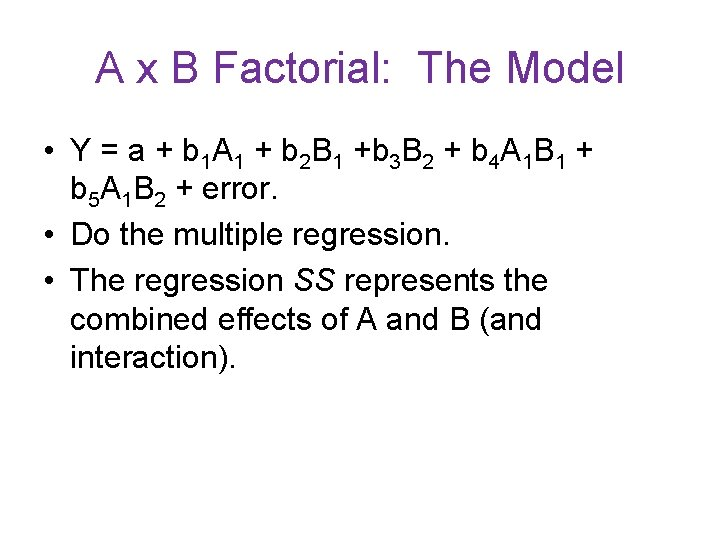A x B Factorial: The Model • Y = a + b 1 A 1 + b 2 B 1 +b 3 B 2 + b 4 A 1 B 1 + b 5 A 1 B 2 + error. • Do the multiple regression. • The regression SS represents the combined effects of A and B (and interaction).Partitioning the Sums of Squares • Drop A 1 from the full model. – The decrease in the regression SS is the SS for the main effect of A. • Drop B 1 and B 2 from the full model. – The decrease in the regression SS is the SS for the main effect of B. • Drop A 1 B 1 and A 1 B 2 from the full model – The decrease in the regression SS is the SS for the interaction.Unique Sums of Squares • This method produces unique sums of square for each effect, representing the effect after eliminating overlap with any other effects in the model. • In SAS these are Type III sums of squares • Overall and Spiegel called them Method I sums of squares.Analysis of Covariance • Simply put, this is a multiple regression where there are both categorical and continuous predictors. • In the ideal circumstance (the grouping variables are experimentally manipulated), there will be no association between the covariate and the grouping variables. • Adding the covariate to the model may reduce the error sums of square and give you more power.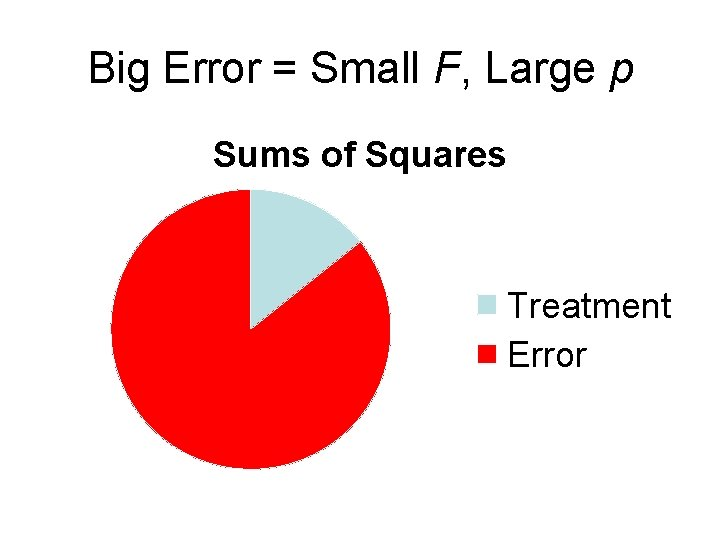Big Error = Small F, Large p Sums of Squares Treatment Error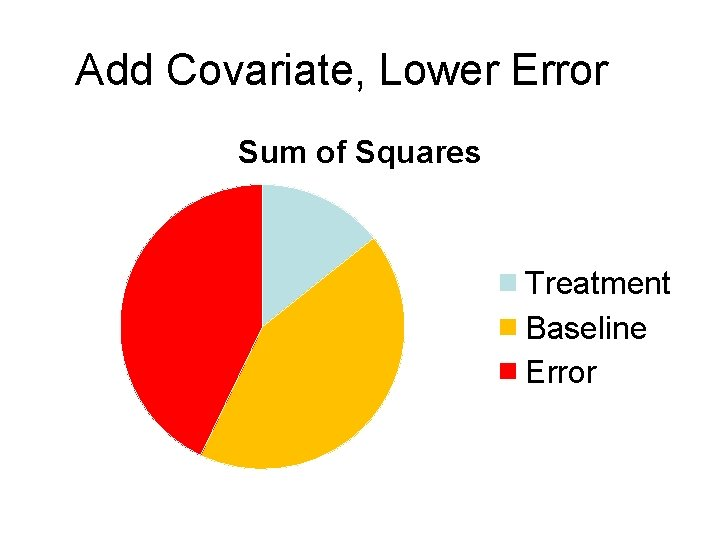Add Covariate, Lower Error Sum of Squares Treatment Baseline ErrorBig F = Happy Researcher Sum of Squares Treatment Baseline Scatophobin Error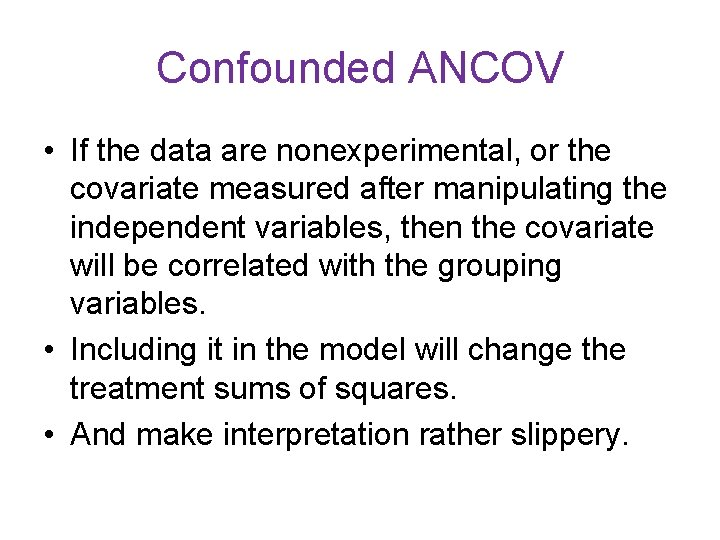Confounded ANCOV • If the data are nonexperimental, or the covariate measured after manipulating the independent variables, then the covariate will be correlated with the grouping variables. • Including it in the model will change the treatment sums of squares. • And make interpretation rather slippery.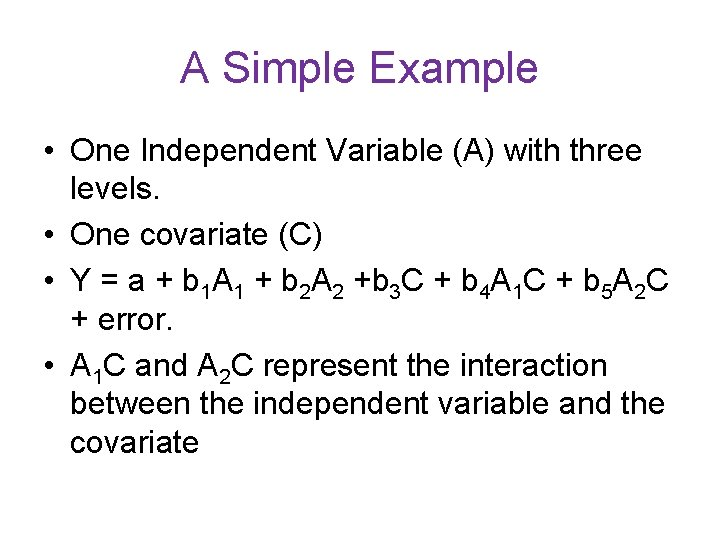A Simple Example • One Independent Variable (A) with three levels. • One covariate (C) • Y = a + b 1 A 1 + b 2 A 2 +b 3 C + b 4 A 1 C + b 5 A 2 C + error. • A 1 C and A 2 C represent the interaction between the independent variable and the covariateCovariate x IV Interaction • We drop the two interaction terms from the model. • If the regression SS decreases markedly, then the relationship between the covariate and Y varies across levels of the IV. • This violates the homogeneity of regression assumption of the traditional ANCOV.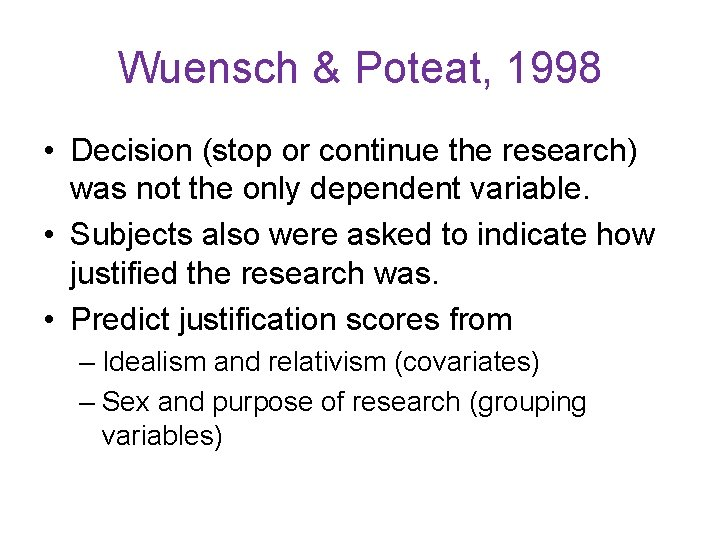Wuensch & Poteat, 1998 • Decision (stop or continue the research) was not the only dependent variable. • Subjects also were asked to indicate how justified the research was. • Predict justification scores from – Idealism and relativism (covariates) – Sex and purpose of research (grouping variables)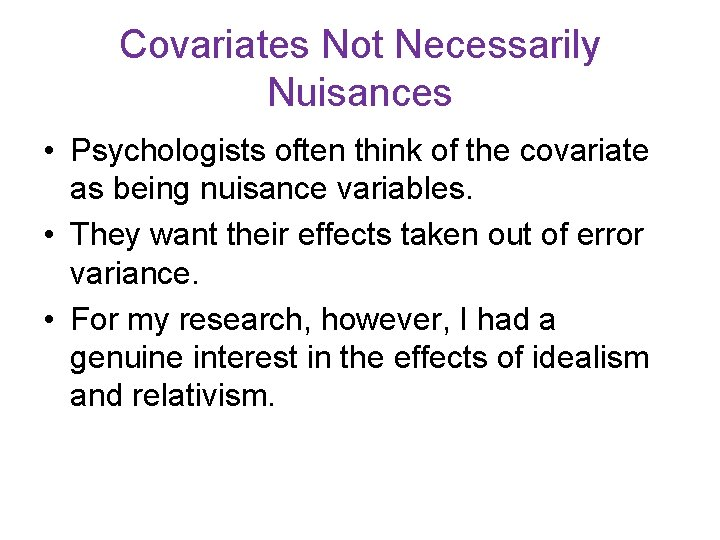Covariates Not Necessarily Nuisances • Psychologists often think of the covariate as being nuisance variables. • They want their effects taken out of error variance. • For my research, however, I had a genuine interest in the effects of idealism and relativism.The Results • There were no significant interactions. • Every main effect was significant. • Idealism was negatively related to justification. • Relativism was positively related to justification. • Men thought the research more justified than did women.• Purpose of the research had a significant effect. • The cosmetic testing and neuroscience theory testing received mean justification ratings significantly less than those of the medical research. • Hmmm, our students think the cosmetic testing not justified, but they vote to continue it anyhow.